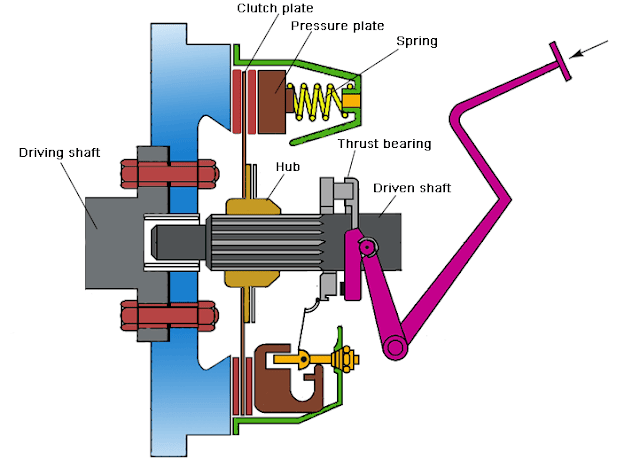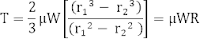Design of Plate Clutch (Uniform pressure theory and uniform wear theory) | Mecholic

# Design of Plate Clutch (Uniform pressure theory and uniform wear theory)Plate clutch is an example of the friction clutch. It consists of two flanges, one of them is rigidly keyed to driving shaft, and the other is free to slide along the driven shaft due to the splined connection. A compression spring is used to press these flanges against each other and torque is transmitted by friction. A suitable mechanism is provided to compress the clutch spring to disengage the clutch.

The amount of torque transmitted depend on axial force, the coefficient of friction, the area of contact.

🔗Energy Loss by Friction Clutch During Engagement
🔗Difference between Single plate clutch and Multi plate clutch

### Design of single plate clutch

T = Torque transmitted
µ = Coefficient of friction
r1, r2 = External and internal radius of contact surface.
p = Intensity of pressure
There are two cases, uniform pressure and uniform wear.

1. Uniform pressure theory
Assuming the pressure is uniformly distributed over the entire surface of friction face. i.e. p = constantMean radius of friction surface,2. Uniform wear theory
Assumption, in this case, pr = constant
p is the normal pressure intensity at a distance r from the axis.Mean radius of friction surface,The intensity of pressure is maximum at the inner radius of friction surface and minimum at the outer radius.
pmax r2= constant
pmin r1= constant

### Design of multi-plate clutch

Fig. shows a multi-plate clutch. A multi-plate clutch is used where large torque is required to be transmitted. They have a number of pairs of contacting surfaces.
For multi-plate clutch
Number of pairs of contacting surface n = n1+n2-1
Here n1 and n2 are the number of discs on the driving and driven shaft respectively.

The total torque transmitted,for uniform pressure theoryfor uniform wear theory